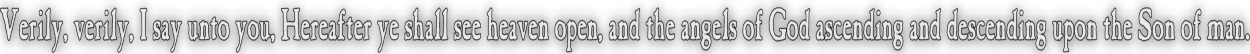None:
Polyps:
Strongs:CreationismLogical NOT There are three "balances" that hold true with a particular choice of oil, say 'd'. {d,f,g} => {e,f,g} <= {d,e,g} (g as wine) {d,f,g} => {e,f,g} <= {d,e,f} (f as wine) {d,e,f} => {e,f,g} <= {d,e,g} (e as wine) Similarly there is a fourth column of the remaining balance that contradicts the choice of oil and wine, namely; {d,f,g} => {e,f,g} <= {d,e,g} (g as wine) contradicted by {d,e,f} = g {d,f,g} => {e,f,g} <= {d,e,f} (f as wine) contradicted by {d,e,g} = f {d,e,f} => {e,f,g} <= {d,e,g} (e as wine) contradicted by {d,f,g} = e Likewise we may add a fourth row (in conflict) to this fourth column from which we recover our initial balances. Namely that constructing a balance from; {d,e,f} = g {d,e,g} = f {d,f,g} = e In any arrangement (with d as wine) is contracdicted by the fourth balance of d = {e,f,g} (With 'd' as oil. The synthesis of all our inital three balances.) Therefore in the method itself, the contradiction of the contradiction of the method supports the method: The negation then ( made by switching the roles of oil and wine) results in the analogy of the logical "NOT" operation. Likewise the process gives the appearance of logic: in the case here that the switch of conjunction and disjunction are logical opposites. In some manner what we have reached is that the result or synthesis if not a possible conjunction, is then logically the disjunction. This sounds like nonsense: But the opposites in view are the thesis/antithesis, and not the synthesis. What is in view is that the fourth balance contradicts the method used, and results in an alternate choice of "wine" for "oil" and vice versa. The effect is an alternate synthesis that has the appearance of "NOT" in that it has the "negation of negation" results with the initial synthesis. If the synthesis itself is false, why not take it (as wine) off the table? Wine becomes oil, and oil becomes wine. It becomes a "negative fact" to be put to one side every bit as much as an absolute truth may also be put to one side to resolve differences in opinion. Remember that the dialectic itself makes no claim that any position (true or false) is a 'fact' to be held in regard. Continue To Next Page Return To Section Start Return To Previous Page'